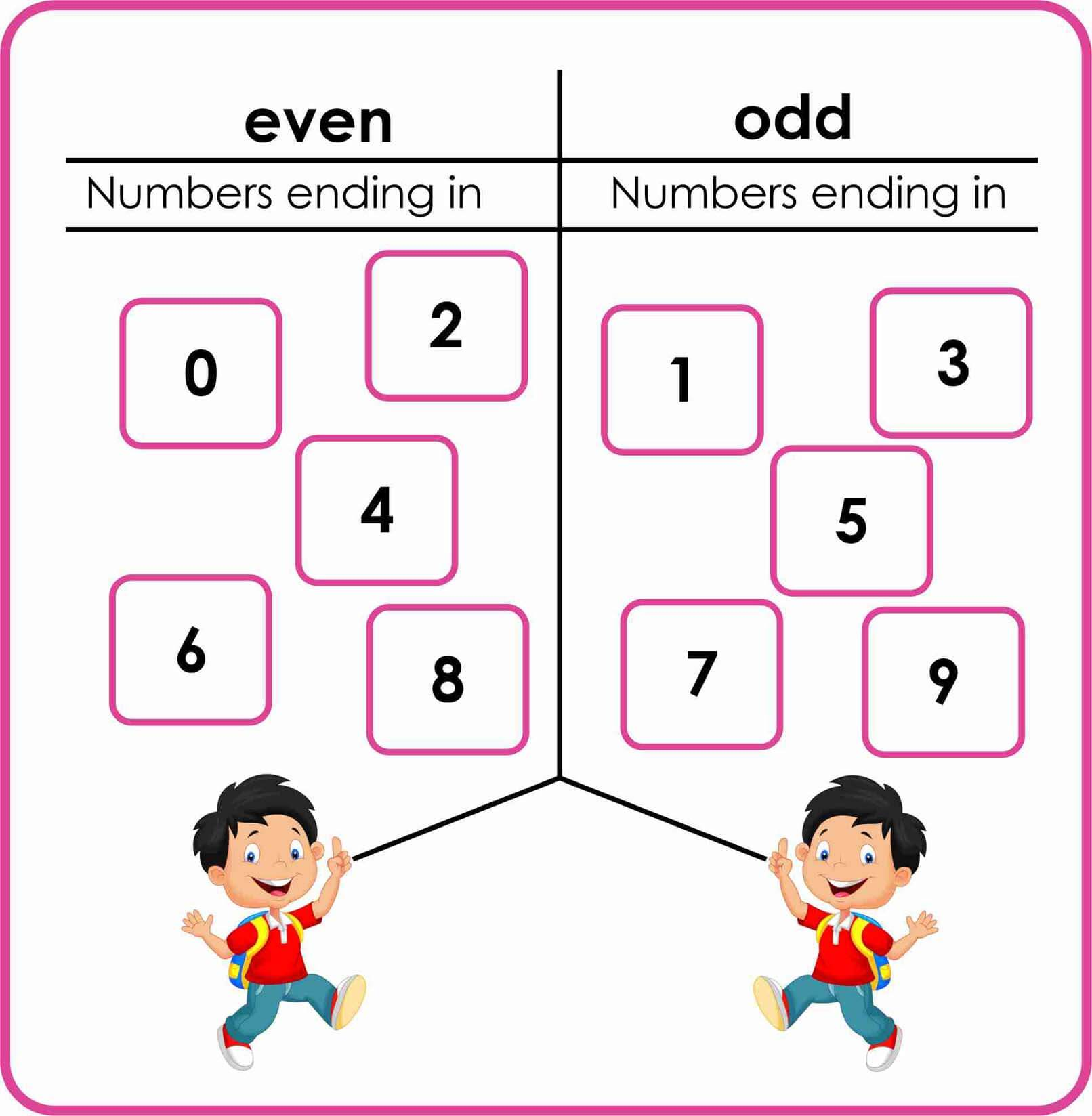# odd and even numbers-worksheetsLooking for a printable odd and even numbers worksheets and charts? Here you can find free worksheets and charts. I have created these odd and even number printable worksheets you can print out these worksheets on this page can be used to teach your children’s about the differences between odd and even numbers. These worksheets also include a wide variety of questions enabling children’s to experience the types of questions that can be framed on the topic. This inevitably allows children’s to get good grades in their exams.

## What is odd Numbers:

However, 13 mangoes cannot be grouped in this way, so 13 is not an even number. An odd number is a number that is not divisible by 2. The remainder, in the case of an odd number is always “1”. 13 is an odd number.

## What is even Numbers :

A number that is divisible by 2 and generates a remainder of 0 is called an even number. Examples of even numbers are 2, 4, 6, 8, 10, etc. For example, assume you have 10 mangoes. These mangoes may be divided into two groups, each having 5 mangoes. So, ten is an even number.

Subtraction:

Multiplication:

## Free Printable odd and even Numbers Worksheets:

Below you can view and download free odd and even numbers worksheets. These worksheets are the perfect way to combine fun with learning to give children’s a holistic studying experience.

If a number divides by 2 entirely, it is an
even number

0 is the smallest even number.

0 is the smallest even number.

If a number does not divide by 2 entirely,
it is an odd number

Double of an odd number is an even
number. For example, 3 × 2 =. 6

Double of an even number is also an even
number. For example, 2 × 2 = 4.

Adding two odd numbers, gives an even
number.

For example, 1 + 3 = 4.

Adding two even numbers, given as even
number. For example,2+ 4 = 6 .

Adding an even number and an odd
number, gives an odd number. For
example,2 + 1=3.

Subtracting two odd numbers, gives an
even number. For example,3 – 1= 2.

Subtractingtwo even numbers, given as
even number. For example,6-2 =4.

Subtracting an odd number from an even
number, gives an odd number. For
example, 6 – 1= 5.

## odd and even numbers chart:

Below you can download odd and even number chart. This table charts show the odd and even numbers. On the charts, the first odd number is 1, the last is 99, and the first even number is 2, the last one is 100.